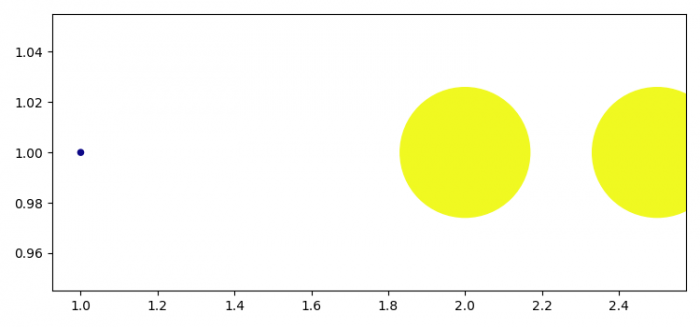# How can I convert from scatter size to data coordinates in Matplotlib?

MatplotlibPythonData Visualization

#### Python Data Science basics with Numpy, Pandas and Matplotlib

Most Popular

63 Lectures 6 hours

#### Data Visualization using MatPlotLib & Seaborn

11 Lectures 4 hours

#### MatPlotLib with Python

9 Lectures 2.5 hours

To convert from scatter size to data coordinates in Matplotlib, we can take the following steps −

• Set the figure size and adjust the padding between and around the subplots.
• Create x and s data points using numpy.
• Create a figure and a set of subplots.
• Make a scatter plot with X and s, cmap and color info.
• To display the figure, use show() method.

## Example

import numpy as np
from matplotlib import pyplot as plt

plt.rcParams["figure.figsize"] = [7.50, 3.50]
plt.rcParams["figure.autolayout"] = True

X = np.array([[1, 1], [2, 1], [2.5, 1]])
s = np.array([20, 10000, 10000])

fig, ax = plt.subplots()

ax.scatter(X[:, 0], X[:, 1], s=s, cmap='plasma', c=s)

plt.show()

## Output